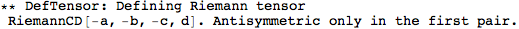xTras Symbol

# SymCovDQ

 SymCovDQ[cd] gives True if cd has been defined as a symmetrizable covariant derivative, and False otherwise. SymCovDQis also an option for DefCovD specifying whether the derivative to be defined is symmetrizable or not.
• The default value for the option SymCovDQ of DefCovD is False.
A symmetrizable covariant derivative can be defined as follows:
Note that the option SymCovDQ could also have been given to DefMetric.
CD is now registered as a symmetrizable covariant derivative:
This means that we can now use multipe incides to indicate symmetrize derivative:
A symmetrizable covariant derivative can be defined as follows:
 In:=Note that the option SymCovDQ could also have been given to DefMetric.
CD is now registered as a symmetrizable covariant derivative:
 In:=
 Out=This means that we can now use multipe incides to indicate symmetrize derivative:
 In:=
 Out=Out=